[First Hit]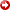The Use of Structural Modelling in the Simulation of Naturally Fractured Reservoirs

By

## Chris McKeown1

Search and Discovery Article #40026 (2001)

1Midland Valley Exploration Ltd (www.mve.com), 14 Park Circus, Glasgow, UK. ([email protected])

Editorial Note: This article, which is highly graphic (or visual) in design, is presented as: (1) four posters, with (a) each represented in JPEG by a small, low-resolution image map of the original; each illustration or section of text on each poster is accessible for viewing at screen scale (higher resolution) by locating the cursor over the part of interest before clicking; and (b) each represented by a PDF image, which contains the usual enlargement capabilities; and (2) searchable HTML text with figure captions linked to corresponding illustrations with descriptions.

First Poster

Second Poster

Third Poster

Fourth Poster

Introduction

Naturally fractured reservoirs are increasingly forming a significant proportion of operators’ portfolios. The flow simulation of such reservoirs requires an understanding of the spatial distribution of reservoir units and their associated petrophysical properties; porosity, permeability, water saturation, relative permeability and capillary pressure. Such information is difficult to obtain away from wells.

tIntroduction

tIntroduction

tIntroduction

tIntroduction

tIntroduction

tIntroduction

tIntroduction

tIntroduction

tIntroduction

Figure Captions

###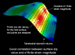Figure 7. Comparison between dip and e1 finite strain magnitude.

####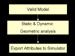Figure 8. Use of results fromgeometricanalyses.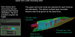Figure 9. Fractures generated between beds.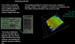Figure 10. Fracture growth controlled by numerical seeds.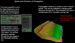Figure 11. Control of fracture propagation.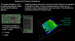Figure 12. Control of fracture impedance.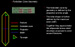Figure 13. Control of stress release ellipsoid shape.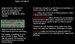Figure 14. Fractures available to be deformed.

### The first stage in the structural modelling workflow is to build a 3D structural model (Figure 1). This can be constructed from all the available data; e.g., 3D seismic surfaces, 2D fault lines (polygons or sticks), 3D fault planes and 2D seismic interpretations. The resultant model is then tested to determine if it is geologically valid. If the model is wrong, then any calculations made with this model will be incorrect.

The next step is to condition the model by splitting the 3D surfaces into fault blocks (constrained by fault data). Then, if required, the structural model is decompacted back to the time of the deposition of the horizon of interest. Once the horizon to be tested is in its temporally correct 3D position, the appropriate restoration algorithm is utilised to flatten the surface to a selected datum.

### The algorithm used is dependent upon the tectonic setting envisaged for the model; e.g., bed parallel slip (associated with compressional regimes) can be accommodated by using flexural slip unfolding. The surface can be unfolded to a pre-selected depth or a palaeosurface (e.g., a palaeobathymetry). Map restoration of the flattened surface is then used to determine if the structural interpretation is geologically valid. If the interpretation is wrong then iteration is required to improve the seismic interpretation. Movement vectors from the map restoration provide information for the kinematic restoration of fault blocks along the fault plane surfaces (Figure 2).

Analysis Workflow

Once a geologically valid model is produced, structural analysis of the model is undertaken (Figure 3). Staticgeometricattributes (e.g., dip, curvature) and dynamicgeometricattributes, such as the evolution of strain, can be output for direct use in calculation of permeability enhancement factors for reservoir simulation.

## StaticGeometricAnalysis

Analysis of staticgeometricparameters such as dip and curvature of surfaces can provide valuable insights into the tectonic evolution of a system. Analysis of the curvature of folded surfaces (Figure 4) yields information on the strain created by deformation and has been shown to provide data on the probable orientation and density of fracturing (Lisle, 1994).

## DynamicGeometricAnalysis

The deformation history of geological system will have a key role in the development of fractures. Once a geological model has been restored to flat (as noted above), forward modelling can be used to track the evolution of the shape of a surface to its present day configuration.

### Finite strain can only be applied to volumes (but can be mapped onto surfaces). The change in position of the vertices of strained, relative to unstrained, tetrahedral allows the calculation of Volumetric Dilatation, Principal Strain Values, Strain Orientations and Plane Strain Ratios (Figure 6).

Strain data can be used to determine the potential for fracture development, fracture density, and the dominant orientation of fractures. It is useful to point out the good correlation between staticgeometricparameters, such as dip, andgeometricparameters, such as the magnitude of maximum strain, (e1).

## Data for Reservoir Simulation

### All of the data derived fromgeometricanalysis can provide attribute maps that can be used to provide insights into reservoir properties in areas of poor well control (Figure 8). For example maps of Gaussian Curvature and Finite Strain azimuths could provide data for conditioning maps of permeability enhancement (PEF) factors away from wells.

Fracture Generation

The techniques presented here illustrate a method of generating stochastic, geologically realistic fracture networks that can form the precursor to simulations of flow through fractured rock. The key benefit of using the method presented here is that a model’s deformation history can be used as a key constraint to fracture growth.

Fractures can be generated: a) using active geological controls; b) at any stage in the evolution of the reservoir, and c) in response to inversion or changes in stress as well as tectonic strain.

Multiple layer-bounded sets can be generated at the same time (Figure 9). Seeds are points of stress concentration that can trigger failure and generate a fracture (Figure 10). If the seed probability is set to be constant, then everywhere on the surface has an equal probability of receiving a randomly dropped seed.

Attribute maps, such as those generated fromgeometricanalysis, can be used to control the seeding of fractures. The number of seeds dropped in on each iteration can be controlled and a multiplier applied. A low multiplier makes the rate of increase in fracturing decrease with progressive deformation.

The propagation potential determines how far and in what direction the fracture will propagate (Figure 11). The step length dictates how far each fracture will propagate in a given iteration. The multiplier controls how that step length varies through time. If the multiplier is below 1, the fracture will propagate by shorter and shorter steps, as it grows longer. Orientation map can be used to control the propagation azimuth of the fracture; i.e., the strike of the actual fracture.

Spatial impedance is how effective the rock is going to be at stopping a fracture from growing (Figure 12). In addition, if a fracture is shut, it will be invisible to other fractures and will exert zero fracture impedance. If the fracture is fully open, the approaching fracture will hit a free surface and stop. The open fracture, therefore, has total impedance. The spatial impedance again can be defined with a constant value across the model or with a map.

The forbidden zone (FZ) simulates the area of stress relief around an active fracture (Figure 13). Aspect ratio gives the length/width relationship and defines the general shape. The maximum width stops the FZspreadingan unrealistic distance from the fracture. This will be a function of rock properties and how far stress and stress relief can be transmitted through a rock.

The projection distance defines the end zones, which are a function of fracture length and the tip angle. The projection length gives the distance ahead of the fracture tip that the stress relief (FZ) extends and the tip angle give the angle of the FZ boundary and the projection line. It defines how rapidly the tip wedge reaches the full FZ width. This will control how far fractures can overlap before the FZs interact and stop a fracture from growing.

Generated fracture networks can be attached as objects and exported to other software to be used as input for fracture/matrix simulation (Figure 14).

## Fractures in Reservoir Simulation

As discussed earlier, maps of permeability enhancement factors can then be used in single porosity flow simulation; where the effective grid block permeability is the product of the matrix and fracture permeability.

### This so-called shape factor is related to the size of the unfractured matrix blocks and is often used as a tuning parameter in history matching against well pressure tests. The dual porosity approach can be fraught with complications, especially the estimation of the shape factor (Bourbiaux, et. al., 1999).

Another approach is to generate discrete fracture networks and use the geometrical interactions of the fractures to determine effective fracture permeability (Oda, 1985).

## References

Bourbiaux, B., Granet, S., Landereau, P., Noetinger, B., Sarda, S., and Sabathier, J. C. (1999) Scaling up matrix.fracture transfers in dual porosity models: theory and application. SPE 56557, presented at the 1999 SPE Annual Conference, Houston, Texas, 3-6 October.

Lisle, R. J. (1994) Detection of zones of abnormal strains in structures using gaussian curvature analysis. AAPG Bulletin, Vol. 78, No. 12, p. 1811-1819.

Oda, M. (1985) Permeability tensor for discontinuous rock masses. Geotechnique, Vol 35, p. 483-495.

Swaby, P. A., and Rawnsley, K. D. (1996) An interactive fracture modelling environment. SPE 36004 presented at the 1996 SPE Petroleum Computer Conference, Dallas, Texas, 2-5 June.

Rawnsley, K., and Wei, L. (2001) Evaluation of a new method to build geological models of fractured reservoirs calibrated to production data. Petroleum Geoscience, Vol. 7, p. 23-33.

Warren J. E., and Root (1963) The behavior of naturally fractured reservoirs. SPE Journal, September 1963, p. 245-255.

Acknowledgements

All of the modelling was performed using 3DMove and the Fracture Generator. All the Team at Midland Valley is thanked for their help in preparing this poster. BP is thanked for its contribution to the development of the Fracture Generator.